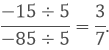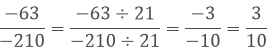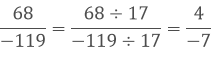×#### Thank you for registering.

One of our academic counsellors will contact you within 1 working day.

Click to Chat

1800-1023-196

+91-120-4616500

CART 0

• 0

MY CART (5)

Use Coupon: CART20 and get 20% off on all online Study Material

ITEM
DETAILS
MRP
DISCOUNT
FINAL PRICE
Total Price: Rs.

There are no items in this cart.
Continue Shopping• Complete JEE Main/Advanced Course and Test Series
• OFFERED PRICE: Rs. 15,900
• View Details

```Chapter 4: Rational Numbers Exercise – 4.4

Question: 1

Write each of the following rational numbers in the standard form:

(i) 2/10

(ii) – 8/36

(iii) 4/-16

(iv) -15/-35

(v) 299/-161

(vi) – 63/- 210

(vii) 68/- 119

(viii) – 195/275

Solution:

(i) The denominator is positive and HCF of 2 and 10 is 2.

Therefore, Dividing the numerator and denominator by 2, we get:

2/10 = 2/2, 10/2 = 1/5

(ii) The denominator is positive and HCF of 8 and 36 is 4.

Therefore, Dividing the numerator and denominator by 4, we get: (- 8)/36

= -8/4, 36/4 = -2/9

(iii) The denominator is negative.

(4x-1)/(-16x-1) = -4/16

HCF of 4 and 16 is 4.

Therefore, Dividing the numerator and denominator by 4, we get: (-4)/4, 16/4

= -1/4

(iv) -15/-35

The H.C.F. of -15 and -35 is 5.

Dividing the Nr and Dr of -15/-35 by 5, we get:(v) 299/-161

The H.C.F. of 299 and -161 is 23.

Dividing the Nr and Dr of 299/-161 by 23, we get:(vi) - 63/-210

The H.C.F of 63 and 210 is 21.

Dividing the Nr and Dr of -63/-210 by 21, we get:(vii) 68/-119

The H.C.F. of 68 and 119 is 17.

Dividing the Nr and Dr of 68/-119 by 17, we get(viii) -195/275

The H. C. F. of 195 and 275 is 5, we get:```### Course Features

• 728 Video Lectures
• Revision Notes
• Previous Year Papers
• Mind Map
• Study Planner
• NCERT Solutions
• Discussion Forum
• Test paper with Video Solution